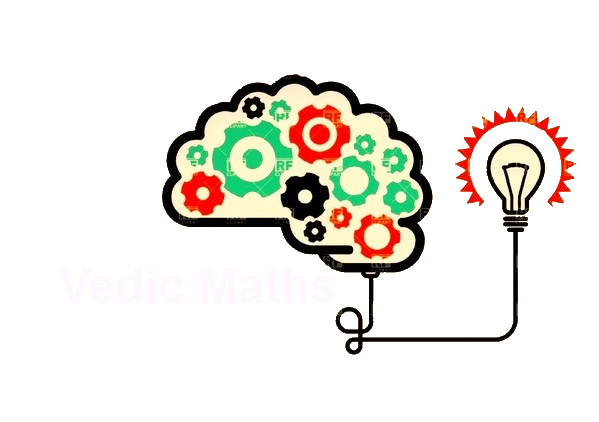• +91-9810395954
• educomiq@gmail.com

# Vedic Math### Vedic Mathematics

Speed Mathematics, also known as Vedic Mathematics, is an ancient Mathematics system of India. This system was rediscovered just half a century ago by Indian Scholar Sri Bharti Krishna Tirthaji. A student who has learnt and practices Speed Math can obtain an intuitive sense of the numbers in a problem and apply the appropriate method to solve the problem efficiently. Computations through Speed Math take a shorter time, quite often in seconds. The benefits one reaps from learning this system is tremendous. This learning is not only useful in academics but also throughout life. Mathematical thinking of a Speed Math learner will be natural with little dependence on a calculator.

• Vedic Math is simpler yet more interesting than regular Math.
• Vedic Mathematics enriches knowledge and understanding of Mathematics, which shows clear links and continuity between different branches of Mathematics.
• Vedic Mathematics methods come as a boon for all competitive exams (such as Management, Banking, Engineering etc.) as mathematic problems can be solved with amazing accuracy and speed.
• Vedic Mathematics being a most natural way of working can be learnt and mastered with ease and in a very short time.
• Vedic Mathematics system also provides a set of independent crosschecking methods.
• The element of choice and flexibility develops clarity of mind and intuition.
• Leads to improvement in mental ability, sharpness, creativity and intelligence.  Problems are reduced to one-line answers.  Reduces dependence on calculators.  Vedic Math is complementary to regular Math taught in schools.  Students learning Vedic Math excel at school.